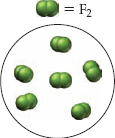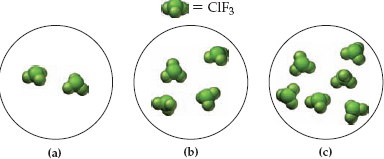×
Get Full Access to Introductory Chemistry - 5 Edition - Chapter 8 - Problem 20p
Get Full Access to Introductory Chemistry - 5 Edition - Chapter 8 - Problem 20p

×

# Chlorine gas reacts with fluorine gas according to the balanced equation:Cl2(g) + 3ISBN: 9780321910295 34

## Solution for problem 20P Chapter 8

Introductory Chemistry | 5th Edition

• Textbook Solutions
• 2901 Step-by-step solutions solved by professors and subject experts
• Get 24/7 help from StudySoup virtual teaching assistantsIntroductory Chemistry | 5th Edition

4 5 1 431 Reviews
26
0
Problem 20P

PROBLEM 20P

Chlorine gas reacts with fluorine gas according to the balanced equation:

Cl2(g) + 3 F2(g) → 2 ClF3(g)If the first figure represents the amount of fluorine available to react, and assuming that there is more than enough chlorine, which figure best represents the amount of chlorine trifluoride that would form upon complete reaction of all of the fluorine?Step-by-Step Solution:

PROBLEM 20P

Chlorine gas reacts with fluorine gas according to the balanced equation:

Cl2(g) + 3 F2(g) ? 2 ClF3(g)If the first figure represents the amount of fluorine available to react, and assuming that there is more than enough chlorine, which figure best represents the amount of chlorine trifluoride that would form upon complete reaction of all of the fluorine?Step by step solution

Step 1 of 2

The balanced chemical equation for the chlorine and fluorine gas is as follows.From the above balanced chemical reaction, one mole of chlorine gas reacts with three moles of fluorine gas to form two moles of chlorine trifluoride.

Step 2 of 2

##### ISBN: 9780321910295

Unlock Textbook Solution